# 10.11. Exercises: Functions¶

If your teacher added you to a Trinket course, complete the exercises there.

Note

The code editors embedded in the exercises all include a link to repl.it in the upper right corner. For the matching Trinkets, click these links:

## 10.11.1. Part A: More Turtles¶

1. Use a loop and the `draw_square` function we wrote in this chapter to draw the image shown below. Make each side 30 units long, and note that the turtle moves away from the last square.

(Check the Turtle Appendix if you need to review the turtle methods).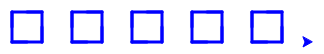2. In the editor above, define a new function called `draw_polygon` that takes 3 parameters—a turtle object, a number of sides, and the length of each side. Place the new function in the lines before `bob = turtle.Turtle()`.

Hint: You drew polygons as part of the Turtle Project in the Loops chapter.

After you finish coding the function, replace the `draw_square` function call in the loop with `draw_polygon` to produce a row of shapes.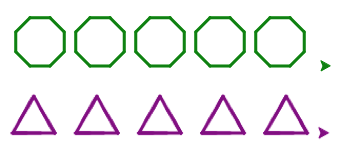Two possible outcomes from using `draw_polygon`.

Try It!

Just for fun, modify your loop code to produce shapes with different colors, sizes, or sides.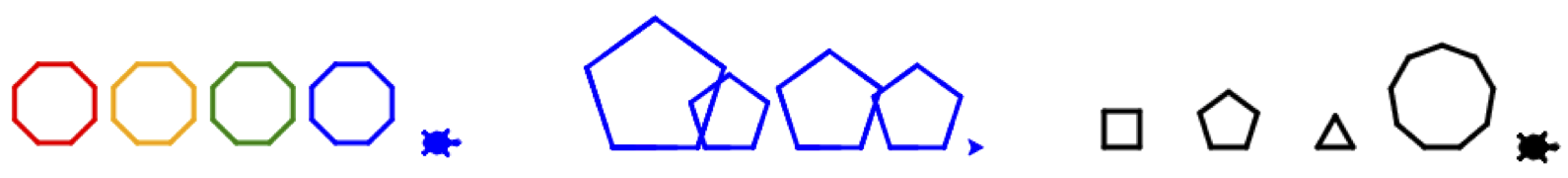3. Write a function called `draw_sprite` that draws a figure like the one shown below. The function needs parameters for the turtle, the number of legs, and the length of the legs.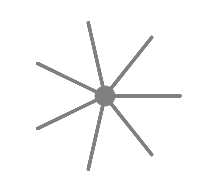Call the function to create a sprite with 10 legs of length 115.

Try It!

Add a parameter to `draw_polygon` called `fancy_corners`. If `True`, then the function should call `draw_sprite` at each corner of the shape. Make the sprite legs half the length of each side.

## 10.11.2. Part B: Return Values¶

1. Write a `shift_case` function that takes a single string parameter and returns a different string. The function should loop through the string and change uppercase characters to lowercase, and lowercase to uppercase.

For example, for the argument `'Hello, World!'`, the function returns `'hELLO, wORLD!'`.

2. Write a `calculate_average` function that uses a list of numbers as the parameter. The function should find and return the average of the numbers from the list. Use the `round()` function to return an average rounded to one decimal place.

Sample results:

1. num_list = [2, 7, 6], average = 5.0

2. num_list = [20, 17, 46, 8], average = 22.8

3. num_list = [0, 3.33, 44, 50, 63, 70.9, 75.2, 83.2], average = 48.7

Note

average = (sum of the elements in list) / (number of elements in list)

3. Write a function `make_line(num_chars, symbol)` that returns a line with exactly `num_chars` symbols. `num_chars` will be an integer, and `symbol` will be a character. Note that the function must RETURN a string, not print it!

If the function call does not provide an argument for `symbol`, use the default character `'#'`.

```print(make_line(5, 'T'))
print(make_line(8))
```

Console Output

```TTTTT
########
```
4. In the editor above, add a function called `make_rectangle` that returns a rectangle string with a given width, height, and symbol. The function should NOT print each row of the rectangle. Instead, it must return a single string that contains the entire rectangle shape.

Tips

1. Call your `make_line` function to create each row of the rectangle string.

2. The newline character, `\n`, will be helpful to you.

3. Do NOT include a newline character at the end of your string.

4. Use `'#'` as the default symbol.

```print(make_rectangle(5, 3))
print(make_rectangle(2, 4, '*'))
```

Console Output

```#####
#####
#####
**
**
**
**
```
5. In the same editor, code a `make_square` function that calls `make_rectangle`. The function should behave something like this:

```print(make_square(4))
print(make_square(3, 'Rutabaga'))
```

Console Output

```####
####
####
####
RutabagaRutabagaRutabaga
RutabagaRutabagaRutabaga
RutabagaRutabagaRutabaga
```

## 10.11.3. Bonus Exercises¶

1. Add a `draw_spiral` function to one of the turtle editors to produce either of the following shapes. Hint: The function needs a turtle, an angle, a starting line length and the number of lines to draw.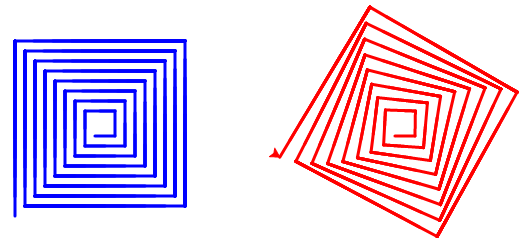The spiral on the left uses an angle of 90°, while the one on the right uses an angle of 89°.

2. Add functions to the editor in part B, exercise 6 to produce any of the following shapes:

```#                       ##
##                     ####
###                   ######
####                 ########
#####               ##########

#
##
###
###
##
#
```